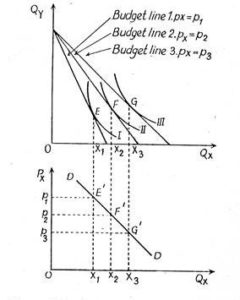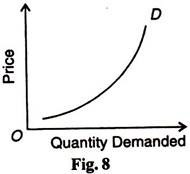# Law of Demand and Demand Curve | Definition, Graph

The compilation of these Consumer Behaviour Notes makes students exam preparation simpler and organised.

## Law of Demand and Demand Curve

The Law of demand is defined as “quantity demand of product decreases if the price of the product increases.” That is if the price of the product rises then the quantity demand falls. Because the opportunity cost of consumer increase which leads consumers to go for any other alternative or they may not buy it. The law of demand and its exceptions are really interesting concepts with many real-life applications. Let us understand.

### Consumer Preference and Demand

Consumer preference theory helps us to understand which combination of two goods a consumer will buy based on the market prices of the goods and subject to a consumer’s budget constraint. What we are interested in, is the amount of a good a consumer actually buys. This is best explained in Microeconomics using the demand function. We will see how to derive the demand function from Indifference curves and what the famous law of demand means.

Derivation of Demand Curve
We know that a consumer maximizes his satisfaction by choosing a bundle of two goods that also falls within his budget, through the IC analysis. We will use this to derive the demand curve for a commodity. Let us consider two goods: X and Y. Let the prices of the two goods are Px and Py and the money income is “M”. The consumer can maximize his utility at the point where his budget line is tangential to the indifference curve. Let this be point ‘E’ in the diagram. The quantity of X consumed is X1.We now vary the price level of good X, keeping the price of good Y and money income constant. Let Px fall. With the same money income, the real purchasing power of the consumer has actually increased. The maximum amount of good X he can buy increases as Px falls since “M” is unchanged. So, the horizontal intercept of the budget line changes (shifts to the right). But the vertical intercept is unchanged because “M” and Py are unchanged. Therefore, the budget line must pivot away from the origin along the horizontal axis as Px falls.

The new budget line is now tangential to a higher IC at point ‘F’. Here, the quantity of X consumed is X 2, which is greater than X1. So, at a lower price of X, a greater quantity of it can be consumed. Again, let the Px fall further. The budget line pivots further away and the new point of tangency becomes G. The quantity of X consumed is X3.

Demand Schedule
We get a Demand schedule that looks like this:Different quantities demanded at varying price levels are given in the schedule above. We can graph these combinations of price and quantity demanded of X. The resulting curve is the Demand Curve of X. It is a graphical representation of various quantities demanded of a commodity at different prices.

### What is the Law of Demand?

Note in figure 1, that the demand curve slopes downwards. This is because as we kept decreasing the price of X, the quantity demanded kept increasing. At a lower price, consumers have a more real income to spend on purchasing the same good, so they can purchase more of it. This leads to a negative relationship between price and quantity demanded. This relation, in economics, is called the Law of Demand. It states that ceteris paribus (other things being equal), “As price falls, the quantity demanded increases and vice versa.”

### Law of Demand Graph### Exceptions to the Law of Demand

There are certain cases in which when the price rises, the quantity demanded also rises (and vice versa). Thus, the law of demand does not apply in these cases. These are termed as exceptions to the law of demand:

• When the good in question is a prestigious good. As the price rises, the quantity demanded must rise for prestige value.
• When the good is a necessity. Even if the price rises, consumption must rise as the good is a necessity.
• An expectation of a further increase in prices causes more to be consumed at current high prices.
• When the good in question is a Giffen good.

Example:

Question:
Is the demand curve of a good always downward sloping?